# Learn How To Use Functions And Methods In Scala

0
2507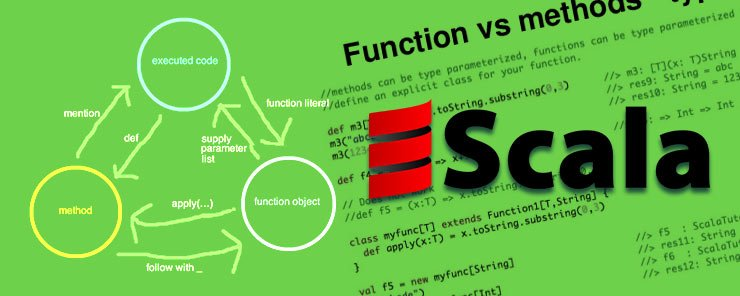Scala provides both methods and functions to organize code and accomplish tasks. Although methods and functions are sometimes used interchangeably to refer to the same thing they are different. Methods belong to a class with a name, signature and annotations while a function is a self contained object that is assignable to a variable. One difference is that methods are invoked on objects unlike functions. With functions you call them and pass arguments while with methods you invoke them on an object and pass arguments. Another difference is that functions can be assigned to variables while methods can not be assigned to variables.

This tutorial assumes you have a Scala development environment set up. If you have not please refer to setting up Scala development environment tutorial. The tutorial also assumes a basic understanding of programing concepts.

Function definition is allowed anywhere within your code and nested functions are also allowed.

Methods in Scala are defined using the def keyword followed by method name, parameter list, data type to be returned and finally the task to be accomplished enclosed by curly braces. The curly braces are used to define a block of code which establishes scope of variables defined within that block. Let us look at an example of a method which given a value in miles converts it to kilometers. To perform this conversion we multiply miles by 1.609. The definition for such a method is shown below

`def mileConverter (miles:Double) : Double = {miles * 1.609}`

Start Scala in interactive mode by running scala at a terminal on ubuntu and define the function above.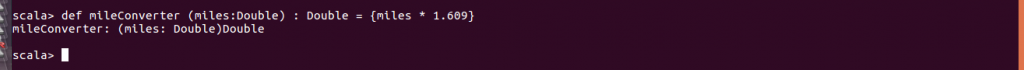Method naming in Scala does not impose restrictions like those found in Java. There are three valid ways to name methods. The first approach allows you to begin with a letter then add letters and digits. You can then choose to add underscores, letters, digits and operators. To demonstrate an example let us repeat definition of method created above

`def mileConverter__++ (miles:Double) : Double = {miles * 1.609}`The second approach to method names is to begin with an operator followed by operators. Repeating the previous example the method name is shown below

`def ++**// (miles:Double) : Double = {miles * 1.609}`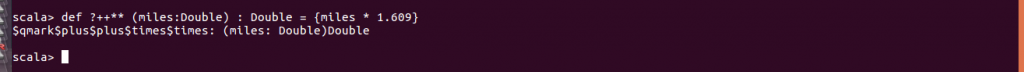The last approach to method naming is to backquote the method name. This is demonstrated below.

```def `miles*1.609` (miles:Double) : Double = {miles * 1.609}
```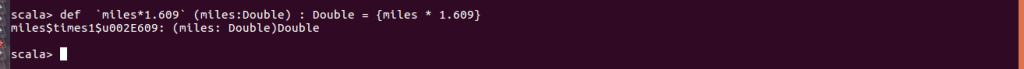Type inference is not supported on arguments so you have to define the argument data types. The return type is inferred but it is good programming practice to specify them. The return statement is used to specify the value to be returned by the method. When omitted the method returns the value of the last statement. When block of code is less than 30 characters you define your function on a single line as demonstrated above. In that case curly braces are not mandatory. When the block of code is longer and has to be on several lines you use a different style. In this case you must use curly braces as shown below.

```def mileConverter (miles:Double) : Double = {
miles * 1.609
}```

Creation of methods with default parameters is also supported. You specify the parameter value after type definition as shown below with space on either side of equals operator.

`def mileConverterdeft (miles:Double = 35) : Double = {miles * 1.609}`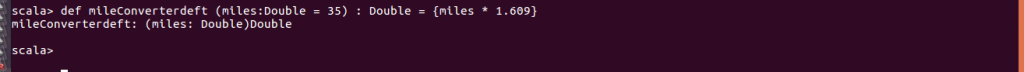Methods without parameters are defined in a similar way as shown below.

`def milestoKm = println("1 mile is equivalent to 1.609 km")`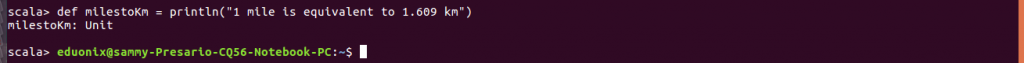To define functions in Scala you specify the arguments and the expression to be applied to arguments using this construct {(arguments) => expression}. This is just one of the many ways you define functions. The curly braces are not mandatory. An example of a function is to convert miles to kilometers is shown below

`(miles: Double) => miles * 1.609`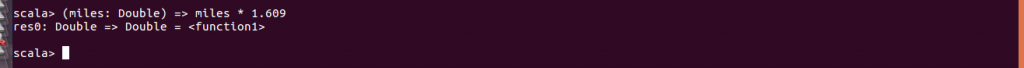It is possible to assign functions to variables. An example is shown below

```val newmiles = (miles: Double) => miles * 1.609
```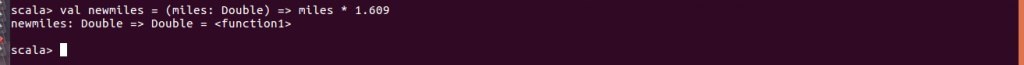This is one of the differences between methods and functions. Whereas functions can be assigned to variables methods cannot. The function above was assigned to a variable newmiles. If we try to assign the method to a variable we get an error. This is demonstrated below

```val milesMethod = def `miles*1.609` (miles:Double) : Double = {miles * 1.609}
```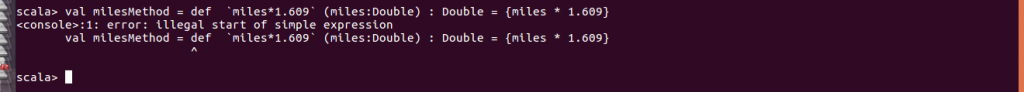Another key difference between methods and functions is that with functions you must have a parameter list even if it is empty. With methods this is not a requirement.

```//method without parameters
def noParams = 23+67```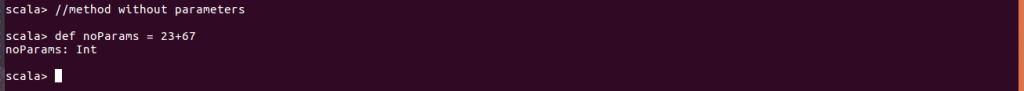```//method with empty params
def emptyParams() = 445```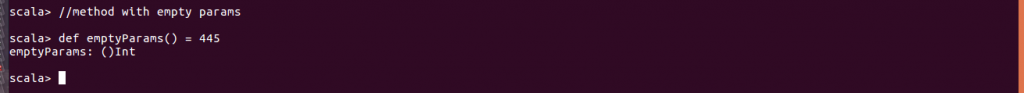```//you cant create a function without params
val wrong = => 500```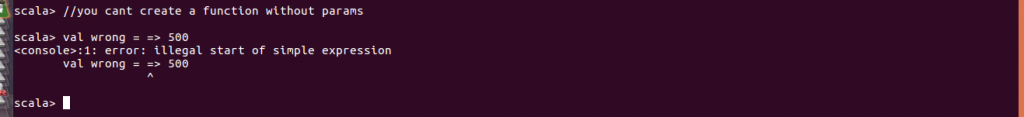```//empty parameter list is allowed in functions
val correct = () => 500```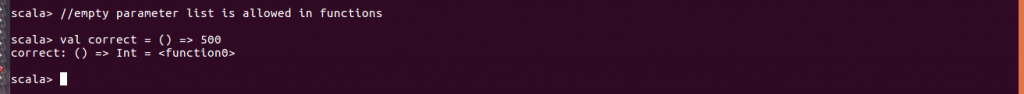When you use a method name you are just invoking it. When you use a function name you are just making a reference to the function. To invoke a function parentheses must be used. Examples of this behavior are shown below using previous function and method.

```//invoke method emptyParams
emptyParams```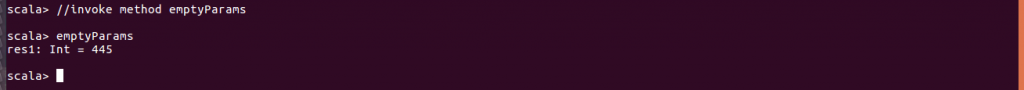```//refer to function correct
correct
```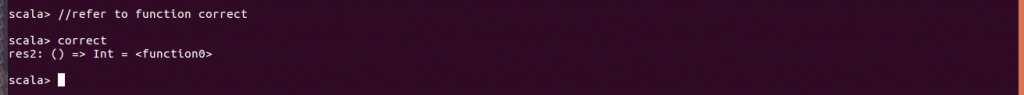```//invoke function correct
correct()```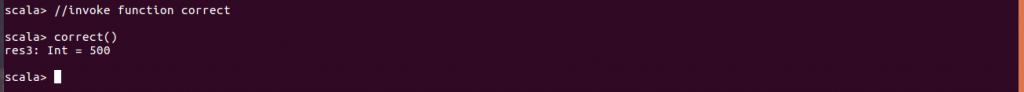Methods and functions have their limitations and strengths which should guide the decision on which to use. There are three areas where functions are limited as compared to methods. When using a function it is not possible to set default values for parameters but this can be done when using a method. Parameterless methods are invoked without parentheses which is impossible with functions. Using parentheses in functions is mandatory even if there are no arguments. Overridden methods in a parent class still allow you access to the parent method. With functions once overriding happens there is no way to use the parent function. You can only use the overridden function.

This tutorial introduced you to functions and methods in Scala. The style of defining functions and methods was discussed and examples to illustrate that were given. Differences between methods and functions were discussed. Finally limitations of using functions as compared to methods were discussed.